#### Discrete problems

First consider adding probabilities to the discrete grid problem of Section 12.2.1. A state is once again expressed as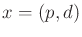. The initial condition is a probability distribution,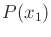, over. One reasonable choice is to makea uniform probability distribution, which makes each direction and position equally likely. The robot is once again given four actions, but now assume that nature interferes with state transitions. For example, if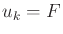, then perhaps with high probability the robot moves forward, but with low probability it may move right, left, or possibly not move at all, even if it is not blocked.

The sensor mapping from Section 12.2.1 indicated whether the robot moved. In the current setting, nature can interfere with this measurement. With low probability, it may incorrectly indicate that the robot moved, when in fact it remained stationary. Conversely, it may also indicate that the robot remained still, when in fact it moved. Since the sensor depends on the previous two states, the mapping is expressed as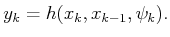(12.19)

With a given probability model,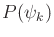, this can be expressed as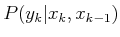.

To solve the passive localization problem, the expressions from Section 11.2.3 for computing the derived I-states are applied. If the sensor mapping used only the current state, then (11.36), (11.38), and (11.39) would apply without modification. However, since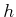depends on both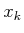and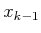, some modifications are needed. Recall that the observations start with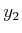for this sensor. Therefore,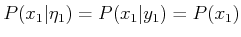, instead of applying (11.36).

After each stage,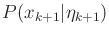is computed from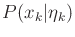by first applying (11.38) to take into account the action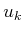. Equation (11.39) takes into account the sensor observation,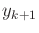, but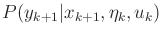is not given because the sensor mapping also depends on. It reduces using marginalization as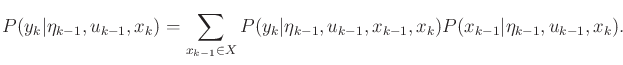(12.20)

The first factor in the sum can be reduced to the sensor model,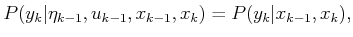(12.21)

because the observations depend only on,, and the nature sensing action,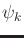. The second term in (12.20) can be computed using Bayes' rule as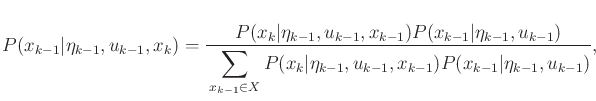(12.22)

in which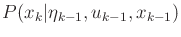simplifies to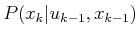. This is directly obtained from the state transition probability, which is expressed as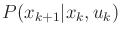by shifting the stage index forward. The term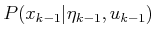is given by (11.38). The completes the computation of the probabilistic I-states, which solves the passive localization problem.

Solving the active localization problem is substantially harder because a search occurs on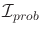. The same choices exist as for the discrete localization problem. Computing an information-feedback plan over the whole I-spaceis theoretically possible but impractical for most environments. The search-based idea that was applied to incrementally grow a directed graph in Section 12.2.1 could also be applied here. The success of the method depends on clever search heuristics developed for this particular problem.

Steven M LaValle 2020-08-14